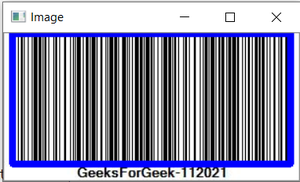Related Articles

# How to Make a Barcode Reader in Python?

• Last Updated : 07 Sep, 2021

Barcode represents data in graphical representation and machine-readable. For making Barcode Reader in Python we are using pyzbar library. Using pyzbar we can decode the one-dimensional barcode and QR-code.

This pyzbar can return 3 fields based on the barcode object:

• Type: There are several kinds of barcodes are available. Which are differentiated by unique code names like CODE-128, Code-11, CODE-39, etc. If the symbol detected by pyzabr then is QRcode for that type is QR-CODE.
• Data: This is data that is embedded inside the barcode. This data is of various kinds ( alphanumerical, numerical, binary, etc..) depending upon the type of barcode.
• Location: This is the collection of points that are located in the code. For barcodes, these points are starting and ending line boundaries. and for QRcode, it is a list of four points corresponding to the four corners of the QR code quad.

For installation:

`pip install pyzbar`

pyzbar Provides the rect method to locate the barcode in the image. Rect stands for a rectangle that will give you the coordinates of the barcode. We can also decode the multiple barcodes included in one image. Using the following steps we are going to make a barcode recorder. (ensure that you are having both libraries installed)

Approach:

• Import cv2.
• Import decode function from pyzbar.
• Take the image from the user.
• Decode that image using pyzbar
• Locate the barcode in the given Image
• Print the data and type of image
• Display located barcode.

Below is the Implementation

## Python3

 `# Importing library``import` `cv2``from` `pyzbar.pyzbar ``import` `decode`` ` `# Make one method to decode the barcode``def` `BarcodeReader(image):``    ` `    ``# read the image in numpy array using cv2``    ``img ``=` `cv2.imread(image)``     ` `    ``# Decode the barcode image``    ``detectedBarcodes ``=` `decode(img)``     ` `    ``# If not detected then print the message``    ``if` `not` `detectedBarcodes:``        ``print``(``"Barcode Not Detected or your barcode is blank/corrupted!"``)``    ``else``:``      ` `          ``# Traverse through all the detected barcodes in image``        ``for` `barcode ``in` `detectedBarcodes: ``          ` `            ``# Locate the barcode position in image``            ``(x, y, w, h) ``=` `barcode.rect``            ` `            ``# Put the rectangle in image using``            ``# cv2 to heighlight the barcode``            ``cv2.rectangle(img, (x``-``10``, y``-``10``),``                          ``(x ``+` `w``+``10``, y ``+` `h``+``10``),``                          ``(``255``, ``0``, ``0``), ``2``)``            ` `            ``if` `barcode.data!``=``"":``              ` `            ``# Print the barcode data``                ``print``(barcode.data)``                ``print``(barcode.``type``)``                ` `    ``#Display the image``    ``cv2.imshow(``"Image"``, img)``    ``cv2.waitKey(``0``)``    ``cv2.destroyAllWindows()` `if` `__name__ ``=``=` `"__main__"``:``  ``# Take the image from user``    ``image``=``"Img.jpg"``    ``BarcodeReader(image)`

Output:

```b'GeeksForGeek-112021'
CODE128```Attention geek! Strengthen your foundations with the Python Programming Foundation Course and learn the basics.

To begin with, your interview preparations Enhance your Data Structures concepts with the Python DS Course. And to begin with your Machine Learning Journey, join the Machine Learning – Basic Level Course

My Personal Notes arrow_drop_up